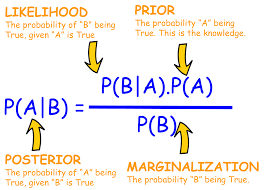Today, I want to dwell in an area non mathematicians’ dread. We all know what probability is and I have discussed this in one of my write-up before, so you might want to do well by looking up my previous writings. What is probability distribution, am sure you are eager to know this and its application to real world problems. In probability theory and statistics, a probability distribution is the mathematical function that gives the probabilities of occurrence of different possible outcomes for an experiment. It is a mathematical description of a random phenomenon in terms of its sample space and the probabilities of events. A sample space is a collection or a set of possible outcomes of a random experiment.

A random variable has a probability distribution, which defines the probability of its unknown values. Random variables can be discrete (not constant) or continuous or both. That means it takes any of a designated finite or countable list of values, provided with a probability mass function feature of the random variable’s probability distribution or can take any numerical value in an interval or set of intervals. Through a probability density function that is representative of the random variable’s probability distribution or it can be a combination of both discrete and continuous.

Two random variables with equal probability distribution can yet vary with respect to their relationships with other random variables or whether they are independent of these. The recognition of a random variable, which means, the outcomes of randomly choosing values as per the variable’s probability distribution function, are called random variates. Probability distribution can either be discrete or continuous. Discrete probability distributions are distribution that can be counted and assume a finite variable. While a continuous probability distributions are those that can be measured.

Few examples of discrete distribution are Bernoulli distribution, Binomial distribution, Poisson distribution, Negative Binomial distribution. These distributions are usually represented by the probability mass functions and the properties are usually denoted by the parameters of such distribution. For example, the parameters of a binomial distributions are usually represented by its number of occurrence usually denoted by n, and the probability of occurrence dented by p. The expected value of a binomial distribution is denoted by np which is also referred to as the mean of the distribution while the variance is denoted by np(1-p). The mean and variance are generally referred to as the first and second moments of the distribution. Other metrices can be calculated from the probability mass function, metrices like moment generating function and cumulant functions can also be computed from the mass function.

For a continuous distribution, they are usually denoted by a probability density function. The following distributions can be categorised under the continuous distribution: Normal distribution, Gamma distribution, Beta Distribution, Log-Normal distribution. We also find the parameters of the distribution. Once the parameters are calculated, we can then calculate the mean and variance of the distribution. For example, a normal distribution has two parameters, and those two parameters can then be used to calculate the mean and distribution of the distribution.

SGD## PROBABILITY – A TOOL FOR DECISION MAKING

in## “MAXIMIZING BALANCE SHEET: UNLOCKING THE PATH TO ORGANIC GROWTH AND FINANCIAL SUCCESS.

in